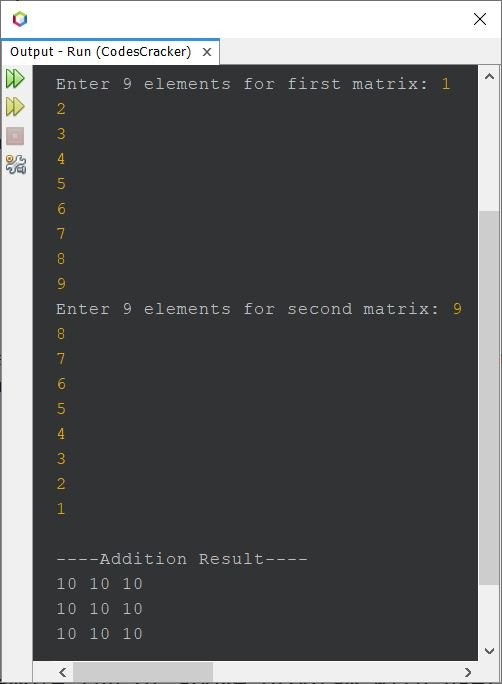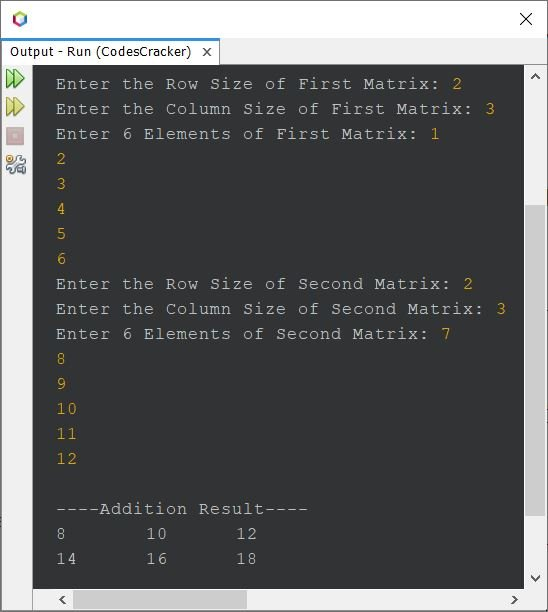# Java Program to Add Two Matrices

This article covers a program in Java, that find and prints the addition result of any two given matrices entered by user at run-time of the program. The first program is created based on 3*3 matrices, whereas the third is created based on m*n matrices.

Then refer to Matrix Addition Rules. Now let's move on, and create a program in Java to add two matrices.

## Addition of Two Matrix in Java using 2D Array

The question is, write a Java program to perform addition of two 3*3 matrices. Elements of both matrices must be received by user at run-time. The program given below is its answer. This program uses 2D array to do the job.

```import java.util.Scanner;

public class CodesCracker
{
public static void main(String[] args)
{
int i, j;
int[][] a = new int;
int[][] b = new int;
int[][] c = new int;

Scanner s = new Scanner(System.in);

System.out.print("Enter 9 elements for first matrix: ");
for(i=0; i<3; i++)
{
for(j=0; j<3; j++)
{
a[i][j] = s.nextInt();
}
}

System.out.print("Enter 9 elements for second matrix: ");
for(i=0; i<3; i++)
{
for(j=0; j<3; j++)
{
b[i][j] = s.nextInt();
}
}

for(i=0; i<3; i++)
{
for(j=0; j<3; j++)
{
c[i][j] = a[i][j] + b[i][j];
}
}

for(i=0; i<3; i++)
{
for(j=0; j<3; j++)
{
System.out.print(c[i][j]+ " ");
}
System.out.print("\n");
}
}
}```

The sample run of above program with user input 1, 2, 3, 4, 5, 6, 7, 8, 9 as nine elements for first, whereas 9, 8, 7, 6, 5, 4, 3, 2, 1 as nine elements for second matrix, is shown in the snapshot given below:The above program, can also be created in this way:

```import java.util.Scanner;

public class CodesCracker
{
public static void main(String[] args)
{
int i, j;
int[][] a = new int;
int[][] b = new int;
int[][] c = new int;

Scanner s = new Scanner(System.in);

System.out.print("Enter 9 elements for first matrix: ");
for(i=0; i<3; i++)
{
for(j=0; j<3; j++)
a[i][j] = s.nextInt();
}

System.out.print("Enter 9 elements for second matrix: ");
for(i=0; i<3; i++)
{
for(j=0; j<3; j++)
{
b[i][j] = s.nextInt();
c[i][j] = a[i][j] + b[i][j];
}
}

for(i=0; i<3; i++)
{
for(j=0; j<3; j++)
System.out.print(c[i][j]+ " ");
System.out.print("\n");
}
}
}```

## Addition of Two Matrices of Specified Orders in Java

Since the previous program is limited to the matrix of 9 elements only. Therefore, I've modified and created a new one, that allows user to define the size of both the matrices too, along with their elements.

```import java.util.Scanner;

public class CodesCracker
{
public static void main(String[] args)
{
Scanner s = new Scanner(System.in);

System.out.print("Enter the Row Size of First Matrix: ");
int rowOne = s.nextInt();
System.out.print("Enter the Column Size of First Matrix: ");
int colOne = s.nextInt();
int[][] matrixOne = new int[rowOne][colOne];

System.out.print("Enter " +rowOne*colOne+ " Elements of First Matrix: ");
for(int i=0; i<rowOne; i++)
{
for(int j=0; j<colOne; j++)
matrixOne[i][j] = s.nextInt();
}

System.out.print("Enter the Row Size of Second Matrix: ");
int rowTwo = s.nextInt();
System.out.print("Enter the Column Size of Second Matrix: ");
int colTwo = s.nextInt();

if(rowOne==rowTwo && colOne==colTwo)
{
int[][] matrixTwo = new int[rowTwo][colTwo];
int[][] matrixThree = new int[rowTwo][colTwo];

System.out.print("Enter " +rowTwo*colTwo+ " Elements of Second Matrix: ");
for(int i=0; i<rowTwo; i++)
{
for(int j=0; j<colTwo; j++)
{
matrixTwo[i][j] = s.nextInt();
matrixThree[i][j] = matrixOne[i][j] + matrixTwo[i][j];
}
}

for(int i=0; i<rowTwo; i++)
{
for(int j=0; j<colTwo; j++)
System.out.print(matrixThree[i][j]+ "\t");
System.out.print("\n");
}
}
else
}
}```

Here is its sample run with user inputs, 2 and 3 as row and column size, 1, 2, 3, 4, 5, 6 as six elements of first matrix. 2 and 3 as row and column size, 7, 8, 9, 10, 11, 12 as six elements of second matrix:#### Same Program in Other Languages

Java Online Test

« Previous Program Next Program »